### Ratio

When you use a RATIO statement, the procedure produces statistics requested by the statistic-keywords in the PROC SURVEYMEANS  statement.

Suppose that you want to calculate the ratio of variable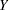to variable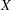. Let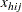be the value of variablefor theth member in clusterin theth stratum.

The ratio oftois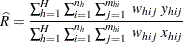PROC SURVEYMEANS  uses the Taylor series method to estimate the variance of the ratio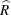as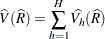where if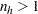,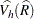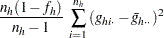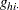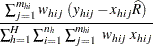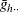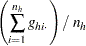and if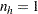,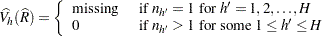The standard error of the ratio is the square root of the estimated variance: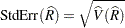When the denominator for a ratio is zero, then the value of the ratio is displayed as '–Infty', 'Infty', or a missing value, depending on whether the numerator is negative, positive, or zero, respectively; and the corresponding internal value is the special missing value '.M', the special missing value '.I', or the usual missing value, respectively.